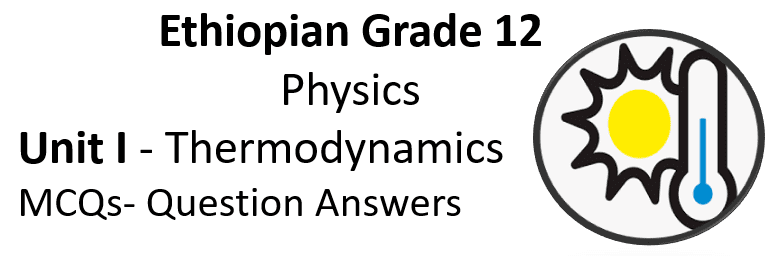Ethiopian Grade 12 Physics Unit I Question Answers (Thermodynamics), will help in EUEE (Grade 12) University Entrance exam. Here is some same multiple choice question answers of Unit 1 Thermodynamics.Unit 1 Thermodynamics

• 1.1 Thermal equilibrium and definition of temperature
• 1.2 Work, heat and the first law of thermodynamics
• 1.3 Kinetic theory of gases
• 1.4 Second law of thermodynamics, efficiency and entropy
• 1.5 Heat engines and refrigerators

Q1. What is thermodynamics?

• A.) study of energy conversion between heat and other forms of energy.
• B.) study of statistical mechanics.
• C.) study of the heat energy which is associated with chemical reactions
• D.) study of the heat energy which is associated with physical transformations

Answers: A.) study of energy conversion between heat and other forms of energy.

Q2. Who was He was the first person to start thinking about how to better the steam engines that were proving so valuable during the Industrial Revolution?

• A.) Isaac Newton
• B.) Joseph Henry
• C.) James Prescott Joule

Q3. What is a mole?

• A.) measurement unit of energy
• B.) measurement of SI unit of amount of substance
• C.) measurement unit of force
• D.) measurement unit of power

Answers: B.)The mole (symbol: mol) is the unit of measurement for the amount of substance in the International System of Units (SI).

Q4. One mole contains exactly ______ elementary entities.

• A.)  1.022 140 76 x 1021
• B.) 3.022 140 76 x 1022
• C.) 6.022 140 76 x 1023
• D.) 8.022 140 76 x 1024

Answers: C.) One mole contains exactly 6.022 140 76 x 1023 elementary entities.

Q5. In which of the following the mole can be used as a measurement unit?

• A.) solid, or
• B.) liquid
• C.) gas
• D.) all of the above

Q6. 1 mol of helium gas contains ______ particles of helium

• A.) 1.02 × 1021
• B.) 2.02 × 1022
• C.) 6.06 × 1023
• D.) 6.02 × 1023

Answers: D.) 1 mol of helium gas contains 6.02 × 1023 particles of helium. This number is also called Avogadro’s number (NA);

Q7. What is molar mass?

• A.) the mass of one mole of a substance.
• B.) the relationship between the number of moles, the number of particles.
• C.) the relationship between one mole and mass.
• D.) All of the above

Answers: A.)It is often helpful to know the mass of 1 mol of a substance. This is called the molar mass.

Q7. Which of the following number(s) is/are required to calculate the mass of the substance?

• A.) moles of a substance
• B.) molar mass
• C.) A and B both
• D.) None of the above

Answers: C.) m = nM (where m = mass of substance, n = number of moles, M = molar mass)

Q8. A block of pure carbon-12 contains 4.2 mol particles, calculate the number of particles in the block.

• A.) 1.5 x 1022 particles
• B.) 2.5 x 1024 particles
• C.) 3.5 x 1026 particles
• D.) 4.5 x 1028 particles

Answers: B) N = NA (Use the relationship between the number of moles and Avogadro’s number)

Q9. A block of pure carbon-12 contains 4.2 mol particles, Find the mass of the block.

• A.) 0.05 kg
• B.) 0.15 kg
• C.) 0.005 kg
• D.) 5.00 kg

Answers: B) m = nM (Use the relationship between number of moles and Molar mass)

Q10. Under which of the following condition in which two bodies are at the same temperature and there is no net transfer of energy between them?

• A.) thermal equilibrium
• B.) zeroth law
• C.) internal energy
• D.) thermal energy

Answers: A)Two physical systems are in thermal equilibrium if there is no net flow of thermal energy between them when they are connected by a path permeable to heat.

Q11. The particles in the hotter body have more ____ energy

• A.) thermal energy
• B.) internal energy
• C.) kinetic energy
• D.) random kinetic energy

Answers: C) On average, the particles in the hotter body have more kinetic energy than those in the colder body.

Q12. Which of the following statement is true?

• A.) Two bodies that are separately in thermodynamic equilibrium with a third body must be in thermodynamic equilibrium with each other.
• B.) Two bodies that are separately in thermal equilibrium with a third body must be in thermal equilibrium with each other
• C.) Two bodies that are separately in hermodynamic equilibrium with a third body must be in thermal equilibrium with each other
• D.) Two bodies that are separately in thermal equilibrium with a third body must be in hermodynamic equilibrium with each other

Answers: B) zeroth law of thermodynamics states it.

Q13. Which of the following is a condition defined as Absolute zero

• A.) 0.00 (K)
• B.) 273.16 (K)
• C.) –273.15 (K)
• D.) None of the above

Answers: A) Absolute zero, the temperature at which all the random motion of particles in a substance ceases, is defined as 0 K.

Q14. No object can reach a temperature of ______  in a finite number of steps.

• A.) Room temperature
• B.) Triple point of water
• C.) Absolute zero
• D.) Ice point

Q15. Which of the following is the critical point of water?

• A.) around 447 K and 20 MPa
• B.) around 547 K and 21 MPa
• C.) around 647 K and 22 MPa
• D.) around 747 K and 23 MPa